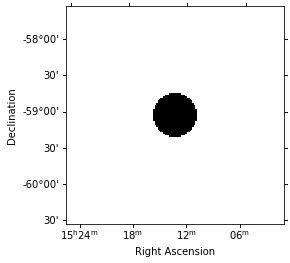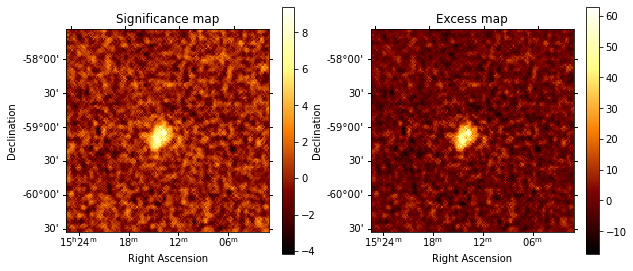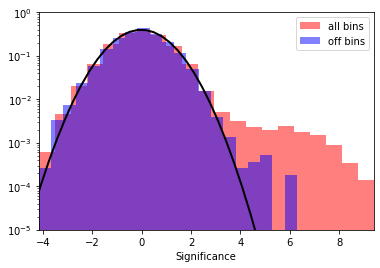This is a fixed-text formatted version of a Jupyter notebook

# Ring background map¶

## Context:¶

One of the challenges of IACT analysis is accounting for the large residual hadronic emission. An excess map, assumed to be a map of only gamma-ray events, requires a good estimate of the background. However, in the absence of a solid template bkg model it is not possible to obtain reliable background model a priori. It was often found necessary in classical cherenkov astronomy to perform a local renormalization of the existing templates, usually with a ring kernel. This assumes that most of the events are background and requires to have an exclusion mask to remove regions with bright signal from the estimation. To read more about this method, see here.

## Objective:¶

Create an excess (gamma-ray events) map of MSH 15-52 as well as a significance map to determine how solid the signal is.

## Proposed approach:¶

The analysis workflow is roughly - Compute the sky maps keeping each observation separately using the Analysis class - Estimate the background using the RingBackgroundMaker - Compute the correlated excess and significance maps using the CorrelatedExcessMapEstimator

The normalised background thus obtained can be used for general modelling and fitting.

## Setup¶

:

%matplotlib inline
import astropy.units as u
from astropy.coordinates import SkyCoord
import matplotlib.pyplot as plt
import numpy as np
from regions import CircleSkyRegion
from scipy.stats import norm

import logging

log = logging.getLogger(__name__)


Now let’s import gammapy specific classes and functions

:

from gammapy.analysis import Analysis, AnalysisConfig
from gammapy.makers import RingBackgroundMaker
from gammapy.estimators import ExcessMapEstimator
from gammapy.maps import Map
from gammapy.datasets import MapDatasetOnOff


## Creating the config file¶

Now, we create a config file for out analysis. You may load this from disc if you have a pre-defined config file.

In this example, we will use a few HESS runs on the pulsar wind nebula, MSH 1552

:

# source_pos = SkyCoord.from_name("MSH 15-52")
source_pos = SkyCoord(228.32, -59.08, unit="deg")

:

config = AnalysisConfig()
# Select observations - 2.5 degrees from the source position
config.observations.datastore = "$GAMMAPY_DATA/hess-dl3-dr1/" config.observations.obs_cone = { "frame": "icrs", "lon": source_pos.ra, "lat": source_pos.dec, "radius": 2.5 * u.deg, }  :  config.datasets.type = "3d" config.datasets.geom.wcs.skydir = { "lon": source_pos.ra, "lat": source_pos.dec, "frame": "icrs", } # The WCS geometry - centered on MSH 15-52 config.datasets.geom.wcs.width = {"width": "3 deg", "height": "3 deg"} config.datasets.geom.wcs.binsize = "0.02 deg" # Cutout size (for the run-wise event selection) config.datasets.geom.selection.offset_max = 3.5 * u.deg # We now fix the energy axis for the counts map - (the reconstructed energy binning) config.datasets.geom.axes.energy.min = "0.5 TeV" config.datasets.geom.axes.energy.max = "5 TeV" config.datasets.geom.axes.energy.nbins = 10 # We need to extract the ring for each observation separately, hence, no stacking at this stage config.datasets.stack = False  :  print(config)  AnalysisConfig general: log: {level: info, filename: null, filemode: null, format: null, datefmt: null} outdir: . observations: datastore:$GAMMAPY_DATA/hess-dl3-dr1
obs_ids: []
obs_file: null
obs_cone: {frame: icrs, lon: 228.32 deg, lat: -59.08 deg, radius: 2.5 deg}
obs_time: {start: null, stop: null}
required_irf: [aeff, edisp, psf, bkg]
datasets:
type: 3d
stack: false
geom:
wcs:
skydir: {frame: icrs, lon: 228.32 deg, lat: -59.08 deg}
binsize: 0.02 deg
width: {width: 3.0 deg, height: 3.0 deg}
binsize_irf: 0.2 deg
selection: {offset_max: 3.5 deg}
axes:
energy: {min: 0.5 TeV, max: 5.0 TeV, nbins: 10}
energy_true: {min: 0.5 TeV, max: 20.0 TeV, nbins: 16}
map_selection: [counts, exposure, background, psf, edisp]
background:
method: null
exclusion: null
parameters: {}
methods: [aeff-default]
parameters: {}
on_region: {frame: null, lon: null, lat: null, radius: null}
containment_correction: true
fit:
fit_range: {min: null, max: null}
flux_points:
energy: {min: null, max: null, nbins: null}
source: source
parameters: {selection_optional: all}
excess_map:
parameters: {}
energy_edges: {min: null, max: null, nbins: null}
light_curve:
time_intervals: {start: null, stop: null}
energy_edges: {min: null, max: null, nbins: null}
source: source
parameters: {selection_optional: all}



## Getting the reduced dataset¶

We now use the config file to do the initial data reduction which will then be used for a ring extraction

:

%%time
# create the config
analysis = Analysis(config)

# for this specific case,w e do not need fine bins in true energy
analysis.config.datasets.geom.axes.energy_true = (
analysis.config.datasets.geom.axes.energy
)

# First get the required observations
analysis.get_observations()

Setting logging config: {'level': 'INFO', 'filename': None, 'filemode': None, 'format': None, 'datefmt': None}
Fetching observations.
No HDU found matching: OBS_ID = 20136, HDU_TYPE = rad_max, HDU_CLASS = None
No HDU found matching: OBS_ID = 20137, HDU_TYPE = rad_max, HDU_CLASS = None
No HDU found matching: OBS_ID = 20151, HDU_TYPE = rad_max, HDU_CLASS = None
No HDU found matching: OBS_ID = 20282, HDU_TYPE = rad_max, HDU_CLASS = None
No HDU found matching: OBS_ID = 20283, HDU_TYPE = rad_max, HDU_CLASS = None
No HDU found matching: OBS_ID = 20301, HDU_TYPE = rad_max, HDU_CLASS = None
No HDU found matching: OBS_ID = 20302, HDU_TYPE = rad_max, HDU_CLASS = None
No HDU found matching: OBS_ID = 20303, HDU_TYPE = rad_max, HDU_CLASS = None
No HDU found matching: OBS_ID = 20322, HDU_TYPE = rad_max, HDU_CLASS = None
No HDU found matching: OBS_ID = 20323, HDU_TYPE = rad_max, HDU_CLASS = None
No HDU found matching: OBS_ID = 20324, HDU_TYPE = rad_max, HDU_CLASS = None
No HDU found matching: OBS_ID = 20325, HDU_TYPE = rad_max, HDU_CLASS = None
No HDU found matching: OBS_ID = 20343, HDU_TYPE = rad_max, HDU_CLASS = None
No HDU found matching: OBS_ID = 20344, HDU_TYPE = rad_max, HDU_CLASS = None
No HDU found matching: OBS_ID = 20345, HDU_TYPE = rad_max, HDU_CLASS = None
No HDU found matching: OBS_ID = 20346, HDU_TYPE = rad_max, HDU_CLASS = None
No HDU found matching: OBS_ID = 20365, HDU_TYPE = rad_max, HDU_CLASS = None
No HDU found matching: OBS_ID = 20366, HDU_TYPE = rad_max, HDU_CLASS = None
No HDU found matching: OBS_ID = 20367, HDU_TYPE = rad_max, HDU_CLASS = None
No HDU found matching: OBS_ID = 20368, HDU_TYPE = rad_max, HDU_CLASS = None
Number of selected observations: 20

CPU times: user 33.9 ms, sys: 2.35 ms, total: 36.3 ms
Wall time: 36.2 ms

:

print(analysis.config)

AnalysisConfig

general:
log: {level: INFO, filename: null, filemode: null, format: null, datefmt: null}
outdir: .
observations:
datastore: \$GAMMAPY_DATA/hess-dl3-dr1
obs_ids: []
obs_file: null
obs_cone: {frame: icrs, lon: 228.32 deg, lat: -59.08 deg, radius: 2.5 deg}
obs_time: {start: null, stop: null}
required_irf: [aeff, edisp, psf, bkg]
datasets:
type: 3d
stack: false
geom:
wcs:
skydir: {frame: icrs, lon: 228.32 deg, lat: -59.08 deg}
binsize: 0.02 deg
width: {width: 3.0 deg, height: 3.0 deg}
binsize_irf: 0.2 deg
selection: {offset_max: 3.5 deg}
axes:
energy: {min: 0.5 TeV, max: 5.0 TeV, nbins: 10}
energy_true: {min: 0.5 TeV, max: 5.0 TeV, nbins: 10}
map_selection: [counts, exposure, background, psf, edisp]
background:
method: null
exclusion: null
parameters: {}
methods: [aeff-default]
parameters: {}
on_region: {frame: null, lon: null, lat: null, radius: null}
containment_correction: true
fit:
fit_range: {min: null, max: null}
flux_points:
energy: {min: null, max: null, nbins: null}
source: source
parameters: {selection_optional: all}
excess_map:
parameters: {}
energy_edges: {min: null, max: null, nbins: null}
light_curve:
time_intervals: {start: null, stop: null}
energy_edges: {min: null, max: null, nbins: null}
source: source
parameters: {selection_optional: all}


:

%%time
# Data extraction
analysis.get_datasets()

Creating reference dataset and makers.
Creating the background Maker.
No background maker set. Check configuration.
Start the data reduction loop.

CPU times: user 9.94 s, sys: 612 ms, total: 10.6 s
Wall time: 10.6 s


## Extracting the ring background¶

Since the ring background is extracted from real off events, we need to use the wstat statistics in this case. For this, we will use the MapDatasetOnOFF and the RingBackgroundMaker classes.

First, we need to create an exclusion mask on the known sources. In this case, we need to mask only MSH 15-52 but this depends on the sources present in our field of view.

:

# get the geom that we use
geom = analysis.datasets.counts.geom
energy_axis = analysis.datasets.counts.geom.axes["energy"]
geom_image = geom.to_image().to_cube([energy_axis.squash()])

regions = CircleSkyRegion(center=source_pos, radius=0.3 * u.deg)For the present analysis, we use a ring with an inner radius of 0.5 deg and width of 0.3 deg.

:

ring_maker = RingBackgroundMaker(
)


### Create a stacked dataset¶

Now, we extract the background for each dataset and then stack the maps together to create a single stacked map for further analysis

:

#%%time
energy_axis_true = analysis.datasets.exposure.geom.axes["energy_true"]
stacked_on_off = MapDatasetOnOff.create(
geom=geom_image, energy_axis_true=energy_axis_true, name="stacked"
)

for dataset in analysis.datasets:
# Ring extracting makes sense only for 2D analysis
dataset_on_off = ring_maker.run(dataset.to_image())
stacked_on_off.stack(dataset_on_off)


This stacked_on_off has on and off counts and acceptance maps which we will use in all further analysis. The acceptance and acceptance_off maps are the system acceptance of gamma-ray like events in the on and off regions respectively.

:

print(stacked_on_off)

MapDatasetOnOff
---------------

Name                            : stacked

Total counts                    : 39183
Total background counts         : 38320.57
Total excess counts             : 862.43

Predicted counts                : 38320.92
Predicted background counts     : 38320.92
Predicted excess counts         : nan

Exposure min                    : 1.11e+09 m2 s
Exposure max                    : 1.30e+10 m2 s

Number of total bins            : 22500
Number of fit bins              : 22500

Fit statistic type              : wstat
Fit statistic value (-2 log(L)) : 26399.35

Number of models                : 0
Number of parameters            : 0
Number of free parameters       : 0

Total counts_off                : 86301192
Acceptance                      : 22500
Acceptance off                  : 49478076



## Compute correlated significance and correlated excess maps¶

We need to convolve our maps with an appropriate smoothing kernel. The significance is computed according to the Li & Ma expression for ON and OFF Poisson measurements, see here. Since astropy convolution kernels only accept integers, we first convert our required size in degrees to int depending on our pixel size.

:

# Using a convolution radius of 0.04 degrees
estimator = ExcessMapEstimator(0.04 * u.deg, selection_optional=[])
lima_maps = estimator.run(stacked_on_off)

:

significance_map = lima_maps["sqrt_ts"]
excess_map = lima_maps["npred_excess"]

:

# We can plot the excess and significance maps
plt.figure(figsize=(10, 10))
ax1 = plt.subplot(221, projection=significance_map.geom.wcs)
ax2 = plt.subplot(222, projection=excess_map.geom.wcs)

ax1.set_title("Significance map")

ax2.set_title("Excess map")

:

<WCSAxesSubplot:title={'center':'Excess map'}, xlabel='Right Ascension', ylabel='Declination'>It is often important to look at the signficance distribution outside the exclusion region to check that the background estimation is not contaminated by gamma-ray events. This can be the case when exclusion regions are not large enough. Typically, we expect the off distribution to be a standard normal distribution.

:

# create a 2D mask for the images
significance_all = significance_map.data[np.isfinite(significance_map.data)]
significance_off = significance_map_off.data[
np.isfinite(significance_map_off.data)
]

plt.hist(
significance_all,
density=True,
alpha=0.5,
color="red",
label="all bins",
bins=21,
)

plt.hist(
significance_off,
density=True,
alpha=0.5,
color="blue",
label="off bins",
bins=21,
)

# Now, fit the off distribution with a Gaussian
mu, std = norm.fit(significance_off)
x = np.linspace(-8, 8, 50)
p = norm.pdf(x, mu, std)
plt.plot(x, p, lw=2, color="black")
plt.legend()
plt.xlabel("Significance")
plt.yscale("log")
plt.ylim(1e-5, 1)
xmin, xmax = np.min(significance_all), np.max(significance_all)
plt.xlim(xmin, xmax)

print(f"Fit results: mu = {mu:.2f}, std = {std:.2f}")

Fit results: mu = -0.02, std = 1.00[ ]:


`